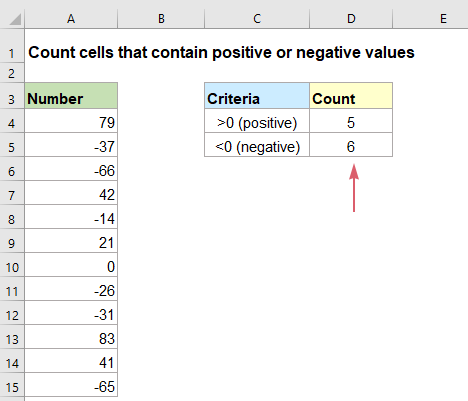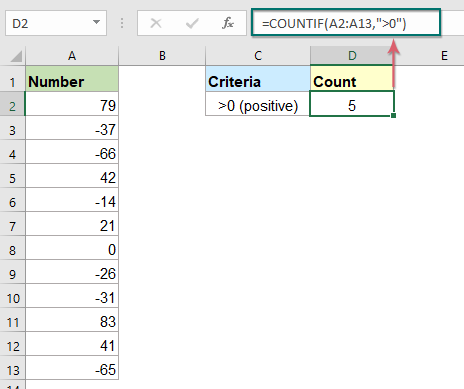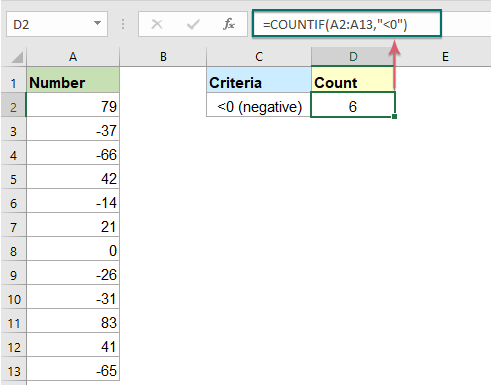## Count number of cells that contain positive or negative values in Excel

To count the number of cells that contain positive or negative numbers from a range of cells in excel, normally, the simple COUNTIF function can do you a favor.#### Count number of cells that contain positive values with COUNTIF function

Here is an easy formula for counting positive numbers in a range of cells, the generic syntax is:

=COUNTIF(range,">0")
• range: The range of cells that contain numbers you want to count;
• “>0”: Indicates the criteria greater than 0.

Please enter or copy the below formula into a blank cell, and then press Enter key to get the result, see screenshot:

=COUNTIF(A2:A13,">0")Tips: If you need to count the cells contain both the positive and zero values, please apply the below formula:

=COUNTIF(A2:A13,">=0")

#### Count number of cells that contain negative values with COUNTIF function

If you want to count the number of negative values in a range, the COUNTIF function also can help you, the generic syntax is:

=COUNTIF(range,"<0")
• range: The range of cells that contain numbers you want to count;
• “<0”: Indicates the criteria less than 0.

Please enter or copy the following formula into a blank cell, and then press Enter key to get the result as below screenshot shown:

=COUNTIF(A2:A13,"<0")#### Relative function used:

• COUNTIF:
• The COUNTIF function is a statistical function in Excel which is used to count the number of cells that meet a criterion.

#### More articles:

• Count number of cells not equal to many values in Excel
• In Excel, you may easily get the number of cells not equal to a specific value by using the COUNTIF function, but have you ever tried to count the cells which are not equal to many values? For example, I want to get the total number of the products in column A but exclude the specific items in C4:C6 as below screenshot shown. This article, I will introduce some formulas for solving this job in Excel.
• Count Number Of Cells That Contain Odd Or Even Numbers
• As we all known, odd numbers have a remainder of 1 when divided by 2, and the even numbers have a remainder of 0 when divided by 2. This tutorial, I will talk about how to get the number of cells that contain odd or even numbers in Excel.
• Count Number Of Cells Contain Numeric Or Non-Numeric Values
• If you have a range of data which contains both numeric and non- numeric values, and now, you may want to count the number of numeric or non-numeric cells as below screenshot shown. This article, I will talk about some formulas for solving this task in Excel.

### The Best Office Productivity Tools

#### Kutools for Excel - Helps You To Stand Out From Crowd

 Popular Features: Find/Highlight/Identify Duplicates  |  Delete Blank Rows  |  Combine Columns or Cells without Losing Data  |  Round without Formula ... Super VLookup: Multiple Criteria  |  Multiple Value   |  Across Multi-Sheets  |  Fuzzy Lookup... Adv. Drop-down List: Easy Drop Down List  |  Dependent Drop Down List  |  Multi-select Drop Down List... Column Manager: Add a Specific Number of Columns  |  Move Columns  |  Unhide Columns  |  Compare Columns to Select Same & Different Cells ... Featured Features: Grid Focus  |  Design View  |  Big Formula Bar  |  Workbook & Sheet Manager  |  Resource Library (Auto Text)  |  Date Picker  |  Combine Worksheets  |  Encrypt/Decrypt Cells  |  Send Emails by List  |  Super Filter  |  Special Filter (filter bold/italic/strikethrough...) ... Top 15 Toolsets:  12 Text Tools (Add Text, Remove Characters ...)  |  50+ Chart Types (Gantt Chart ...)  |  40+ Practical Formulas (Calculate age based on birthday ...)  |  19 Insertion Tools (Insert QR Code, Insert Picture from Path ...)  |  12 Conversion Tools (Numbers to Words, Currency Conversion ...)  |  7 Merge & Split Tools (Advanced Combine Rows, Split Cells ...)  |  ... and more

Kutools for Excel Boasts Over 300 Features, Ensuring That What You Need is Just A Click Away...

Supports Office/Excel 2007-2021 and 365   |   Available in 44 Languages   |   Easy to Uninstall Completely#### Office Tab - Enable Tabbed Reading and Editing in Microsoft Office (include Excel)

• One second to switch between dozens of open documents!
• Reduce hundreds of mouse clicks for you every day, say goodbye to mouse hand.
• Increases your productivity by 50% when viewing and editing multiple documents.
• Brings Efficient Tabs to Office (include Excel), Just Like Chrome, Edge and Firefox.No ratings yet. Be the first to rate!
This comment was minimized by the moderator on the site
gan kalo mau jumlahkan angka yang positif di dalam table rumus nya apa ya gan
This comment was minimized by the moderator on the site
Hello, meisia,
To sum all positive numbers, please use the below formula:
``= SUMIF(B2:B8, ">0")``

To sum all negative numbers, please use the below formula:
``= SUMIF(B2:B8, "<0")``
There are no comments posted here yet